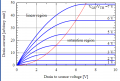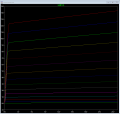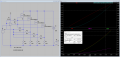# MOSFET Drain Source resistance

#### subatomic particle

Joined May 8, 2018
76
Hello,
when is the Drain Source Resistance Low and when is it high?
When does a MOSFET work as a current source and when does it work as an active load?

#### Irving

Joined Jan 30, 2016
3,300
•subatomic particle

#### BobTPH

Joined Jun 5, 2013
6,268
A MOSFET does act somewhat like a current source when it is in saturation mode. For any given gate voltage, there is a max current after which the current increases very little with increase in Vds.

Bob

#### Irving

Joined Jan 30, 2016
3,300
A MOSFET does act somewhat like a current source when it is in saturation mode. For any given gate voltage, there is a max current after which the current increases very little with increase in Vds.

Bob
Hmmm - when configured in a constant current configuration I suppose you could say its a current source, but that's not the MOSFET, that's the circuit configuration. It isn't a current source in the sense that a battery is a current source.

#### Audioguru again

Joined Oct 21, 2019
5,546
Is the load actually 0.75milli-ohms which is only 0.00075 of an ohm?

The Mosfet needs a Vgs of 10V to fully turn on and the minimum opamp output voltage will be 11.2V then the output current will be (11.2V - 10V)/0.00075= 1600A (!) and explode it and the battery if the input voltage is at the maximum input for the opamp of 12V - 1.5V= 10.5V.

If the load is actually 0.75 of an ohm then the output current will be 667mA.

#### crutschow

Joined Mar 14, 2008
31,477
when configured in a constant current configuration I suppose you could say its a current source, but that's not the MOSFET, that's the circuit configuration.
Well, the added circuitry controlling the MOSFET does help in establishing and stabilizing the current-limit, but the MOSFET makes a pretty good current-source by itself if operated in the saturation region (below), as can be seen by the near constant current for a change in the drain-source voltage.#### Irving

Joined Jan 30, 2016
3,300
Is the load actually 0.75milli-ohms which is only 0.00075 of an ohm?

The Mosfet needs a Vgs of 10V to fully turn on and the minimum opamp output voltage will be 11.2V then the output current will be (11.2V - 10V)/0.00075= 1600A (!) and explode it and the battery if the input voltage is at the maximum input for the opamp of 12V - 1.5V= 10.5V.

If the load is actually 0.75 of an ohm then the output current will be 667mA.
OK, that might have been a bad choice of example, it was a WIP on another project and I picked the first diagram with a single MOSFET as an example confi. For completeness here are the LTSpice generated curves for that device for Vgs = 4.15 - 4.85V:I don't have the actual real device curves to hand but they were remarkably similar - better than I expected.

Here is the actual circuit I'm testing, though right now the heatsink isn't big enough to go to 40A @ 28.8v design spec at 10m/s air flow. I'll probably end up using two of the heatsinks I have now and 6 MOSFETs in total (at £28 each I'd rather not fry them too often). The sense resistors are 50A/75mV ammeter shunts - a lot cheaper and easier to use than PCB mounted devices - I was originally using 100A/75mV ones as I had a couple to hand but they are a little insensitive at low currents.This works pretty much as per the simulation though it drifts off a little as the devices heat up - only to be expected. Right now I'm playing with ideas to select 1, 2 or 4 MOSFETs and changing loop gain to make it a little more flexible at lower currents, say <20A and <10A,the approx limits for 2 and a single MOSFET.

Even so, a 16bit DAC on 3.3v full range gives a (noisy) resolution of around 20mA though realistically I'd say 200mA steps are more practical for all 4 MOSFETs which is fine for my immediate needs.